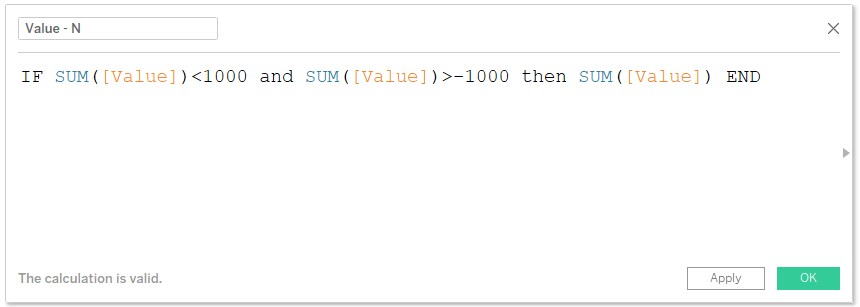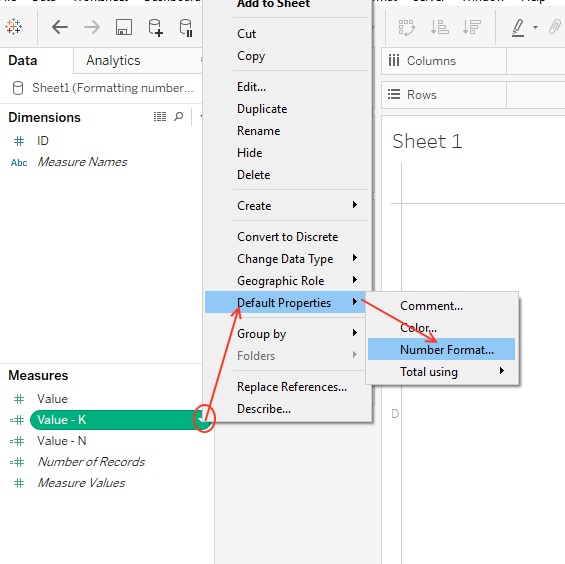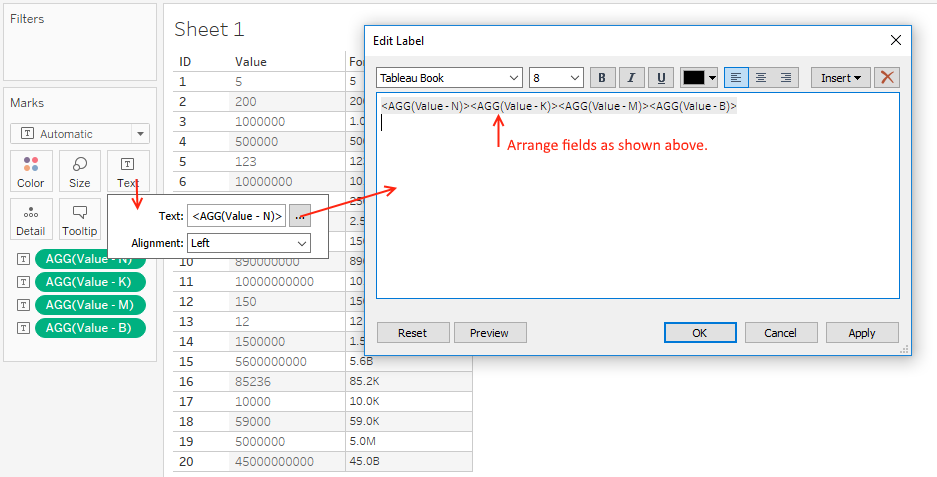Search
• Bernard

# Tableau Tips: Dynamic formatting of values

Updated: May 22In the series Tableau tips, we strive to explore on small hacks which could greatly impact on our dashboards if implemented well. In this article, we'll look forward to solve one of design challenges when aggregating values in Tableau. Imagine of a scenario whereby you're dealing with a set of values ranging from smaller numbers, lets say 5 to billions. Dealing with this range of values can be quite challenging especially when space is limited within your dashboard. As an analyst, its your goal to ensure every important metric is communicated in the limited space and therefore devising a way to deal with this design itch could help a lot. A simple way is formatting values by adding the necessary suffixes as shown in the Table below under 'Formatted values'.This ensures that;

• The numbers occupy smaller space and therefore can be easily used in limited space of the dashboard.

• This resolves the future number challenge, whereby numbers could go beyond the expectation by ensuring all incoming data is formatted by the formula to figures that can fit in the dashboard.

But how can one achieve this in Tableau? Using the same data above here, I'll explore a simple technique of formatting your current data and incoming data using the few steps below. But first, connect the data to Tableau app.

### Step 1: Create Value -N

This consist of values less than 1,000 and won't need to be formatted at all. Note, we've included the negative values in our calculation to take care of the negative values just in case.### Step 2: Create Value -K

This consist of values greater than 999 but less than a million, and will be formatted with the suffix K. (Note, we've included the negative values in the calculation to deal with the negative values just in case).Format Step 2; as shown below.### Step 3: Creating Value -M

This field consist of values greater than or equal to one million but less than a billion to be formatted using suffix M. (Note, our calculation takes care of the the negative values in case they are there).Format Step 3; This can be executed by following the guide on Format Step 2 above, Note to use 'Millions (M)' under 'Display Units'.

### Step 4: Creating Value - B

This field consist of values greater than or equal to a billion and will be formatted with the suffix B, Our assumption is, the highest value in our hypothetical data we can have is in the range of billions. However the same technique can be extended to format higher numbers.Format Step 4; This can be executed by following the guide on Format Step 2 above, Note to use 'Billions (B)' under 'Display Units'.

### Step 5: Lets build a simple chart to put our formulas in a real test.

This can be done by the following;

• Drag the dimension field 'ID' to the Row(s) shelf.

• Next drag the measure field 'Values' to the dimension area, then drag it to the Row(s) shelf next to the field 'ID'.

• Drag all the new calculated fields (Value- N,Value- K,Value- M,Value- B) to the 'Text' tab under the Marks card.

Arrange the fields as shown below.Executing above we've.Note, the same technique can be extended to the tool-tips, by dragging the Calculated fields to the Tool-tip or detail shelf under marks card. Then re-arrange the same under tool-tip as shown below.Executing this, ensures the calculated field is effected on the tool-tip. Simple, isn't?